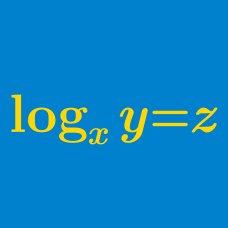Algebra

# Logarithmic Functions: Level 4 Challenges

$\log(\tan 1^\circ ) + \log (\tan 2^\circ) + \log (\tan 3^\circ) + \ldots + \log (\tan 89^\circ ) = \ ?$

Find the sum of the roots of the equation

$(\log_3x)(\log_4x)(\log_5x) = (\log_3x)(\log_4x) +(\log_4x)(\log_5x) +(\log_3x)(\log_5x).$

It is given that $\log_{6}a + \log_{6}b + \log_{6}c = 6$, where $a$, $b$ and $c$ are positive integers that form an increasing geometric sequence and $b - a$ is the square of an integer.

Find $a + b + c.$

The value of $\displaystyle{x}$ that satisfies the equation $\log_{2}(2^{x-1} + 3^{x+1}) = 2x - \log_{2}(3^x)$ can be expressed as $\displaystyle{\log_{a}b}$. What is the value of $\displaystyle{a+b}$?

$\quad$ Note: $a$ and $b$ are both fractions and their sum is an integer.

$\large \log_2(\log_{2^x}(\log_{2^y}(2^{1000}))) = 0$

If $x$ and $y$ are positive integers satisfying the equation above, then find the sum of all possible values of $x+y$.

×